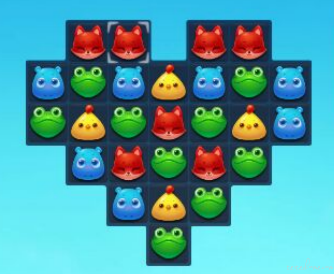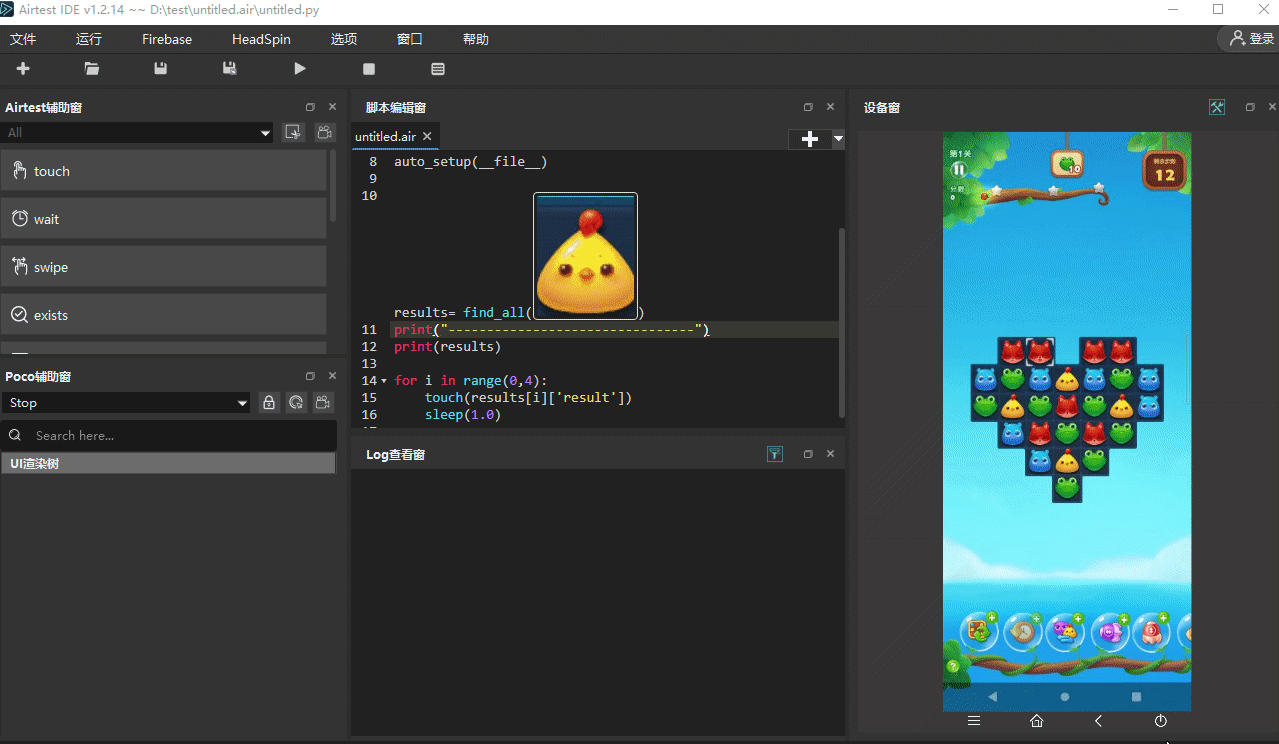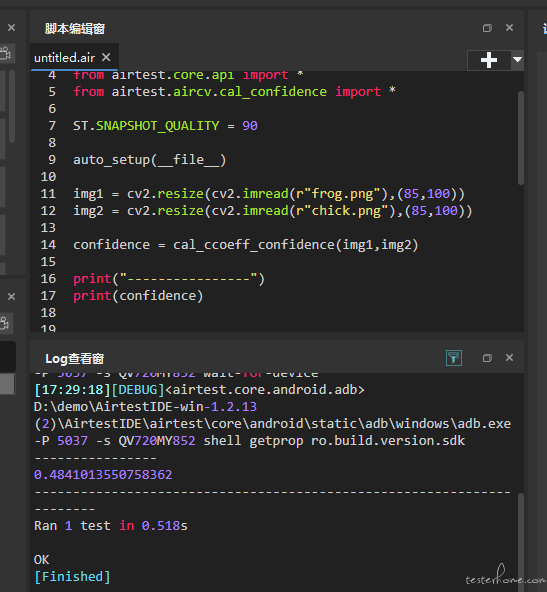# AirtestProject Airtest 的多图查找与两图对比

fishfish-yu · 2022年09月13日 · 最后由 Joo 回复于 2022年09月13日 · 5101 次阅读

### 2. 多图查找：`find_all```````# -*- encoding=utf8 -*-
__author__ = "AirtestProject"

from airtest.core.api import *
auto_setup(__file__)

results= find_all(Template(r"tpl1662624311911.png", record_pos=(-0.002, -0.178), resolution=(1080, 2520)))
print("--------------------------------")
print(results)

for i in range(0,4):
touch(results[i]['result'])
sleep(1.0)
``````

`find_all` 给我们返回的结果是一个列表，列表里面包含了识别结果的字典，从字典的 `result` 键值中，我们可以拿到我们想要的坐标信息：

``````[{'result': (538, 1423), 'rectangle': ((490, 1363), (490, 1483), (587, 1483), (587, 1363)), 'confidence': 0.9852163195610046},
{'result': (301, 1186), 'rectangle': ((253, 1126), (253, 1246), (350, 1246), (350, 1126)), 'confidence': 0.9825006723403931},
{'result': (775, 1186), 'rectangle': ((727, 1126), (727, 1246), (824, 1246), (824, 1126)), 'confidence': 0.9197724461555481},
{'result': (249, 1894), 'rectangle': ((201, 1834), (201, 1954), (298, 1954), (298, 1834)), 'confidence': 0.7261688709259033}]
``````

### 3. 两图对比``````# -*- encoding=utf8 -*-
__author__ = "AirtestProject"

from airtest.core.api import *
from airtest.aircv.cal_confidence import *

auto_setup(__file__)

confidence = cal_ccoeff_confidence(img1,img2)

print("----------------")
print(confidence)
``````

• `cv2.resize` 用于统一图片尺寸，上图中的 (85,100) 为我们指定的目标尺寸
• `cv2.imread`用于读取本地路径下的图片
• `cal_ccoeff_confidence`用于对比两张图片的相似度，返回可信度的值